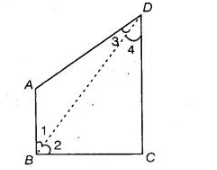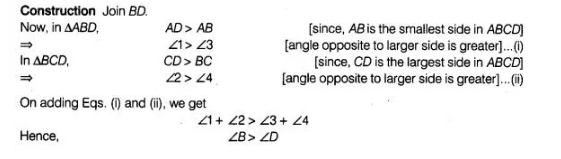# If AB and CD are the smallest and`
Question:

If AB and CD are the smallest and largest sides of a quadrilateral ABCD, out of ∠B and ∠D decide which is greater.

Solution:

Given In quadrilateral $A B C D, A B$ is the smallest and $C D$ is the largest side To find $\angle B>\angle D$ or $\angle D>\angle B$.